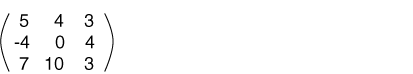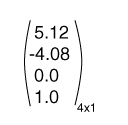The number of rows == the number of columns

# Square Matrix

A square matrix has the same number of rows as columns. In computer graphics, square matrices are used for transformations.A rectangular matrix is one where the number of rows or columns may not be the same. (Some books require that the number of rows and number of columns be different.)

A column matrix consists of a single column. It is a N x 1 matrix. These notes, and most computer graphics texts, use column matrices to represent geometrical vectors. Below is a 4 x 1 column matrix.A row matrix consists of a single row. (Some books use row matrices to represent geometrical vectors).

Some books call a column matrix a column vector and call a row matrix a row vector. This is OK, but can be ambiguous. In these notes the word vector will be used for a geometric object. In these notes, column matrices will be used to represent vectors and will also be used to represent geometric points.

### QUESTION 4:

What are square matrices used for?